Module 10 - Answers Lesson 1 Answer 1 10.1.1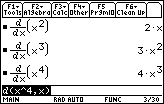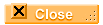Answer 2 10.1.2 The exponent in the function becomes the coefficient of the derivative and the exponent of the derivative is one less than the exponent in the function.Answer 3 10.1.3 Predict that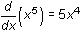and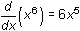, which are verified in the screen below.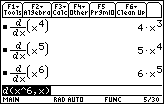Answer 4 10.1.4 Generalize that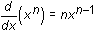, which is verified in the screen below.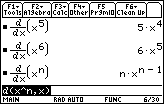Answer 5 10.1.5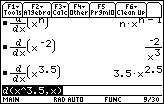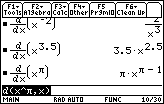The Power Rule appears to be valid for other types of exponents.Lesson 2 Answer 1 10.2.1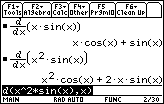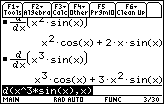Answer 2 10.2.2 Predict that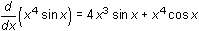, which is verified below.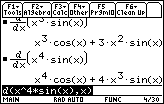Answer 3 10.2.3 Predict that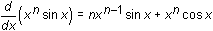.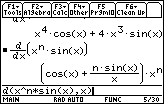Answer 4 10.2.4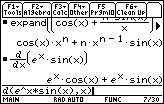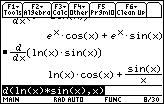Answer 5 10.2.5 Predict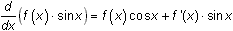, which is equivalent to the expression shown in the screen below.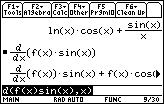Answer 6 10.2.6 Predict that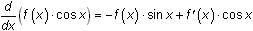and that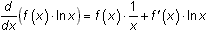, which are each respectively equivalent to the expressions shown below.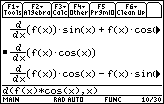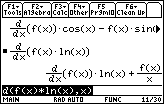Answer 7 10.2.7 The derivative of the product of two functions f and g is given by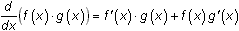.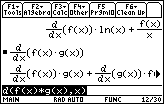You will have to scroll to the right in the History Area to see the entire result of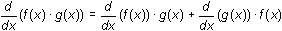, which is equivalent to the one stated in the theorem.Lesson 4 Answer 1 10.4.1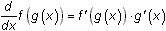This result is known as the Chain Rule for derivatives.Self Test Answer 1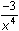Answer 2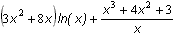Answer 3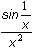Answer 4 2:Save Copy As... in the F1:Tools menu Answer 5 8:Text Editor in the APPs menu, then select 2:open... Answer 6 To find the derivative of a cofunction, negate the derivative of the original function and replace the functions in the derivative with their cofunctions.©Copyright 2007 All rights reserved. | Trademarks | Privacy Policy | Link Policy